Students can Download Tamil Nadu 11th Chemistry Model Question Paper 3 English Medium Pdf, Tamil Nadu 11th Chemistry Model Question Papers helps you to revise the complete Tamilnadu State Board New Syllabus, helps students complete homework assignments and to score high marks in board exams.

## TN State Board 11th Chemistry Model Question Paper 3 English Medium

General Instructions:

1. The question paper comprises of four parts.
2. You are to attempt all the parts. An internal choice of questions is provided wherever applicable.
3. All questions of Part I, II, III and IV are to be attempted separately.
4. Question numbers 1 to 15 in Part I are Multiple Choice Questions of one mark each.
These are to be answered by choosing the most suitable answer from the given four alternatives and writing the option code and the corresponding answer
5. Question numbers 16 to 24 in Part II are two-mark questions. These are to be answered in about one or two sentences.
6. Question numbers 25 to 33 in Part III are three-mark questions. These are to be answered in about three to five short sentences.
7. Question numbers 34 to 38 in Part IV are five-mark questions. These are to be answered in detail Draw diagrams wherever necessary.

Time: 2:30 Hours
Maximum Marks: 70

PART – I

Answer all the Questions: [15 × 1 = 15]
Choose the most suitable answer from the given four alternatives.

Question 1.
40 ml of methane is completely burnt using 80ml of oxygen at room temperature. The volume of gas left after cooling to room temperature. The volume of gas left after cooling to room temperature is …………………….
(a) 40 ml of CO2 gas
(b) 40 ml of CO2 gas and 80 ml of H2O gas
(c) 60 ml of CO2 gas and 60 ml H2O gas
(d) 120 ml of CO2 gas
Solution:
CH4(g) + 2O2(g) → CO2(g) + 2H2O(l)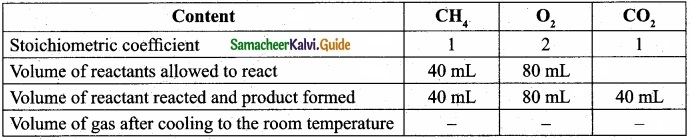Since the product was cooled to room temperature, water exists mostly as liquid. Hence, option (a) is correct.
(a) 40 ml of CO2 gasQuestion 2.
What are the values of n, l, m and s for 3px electron?
(a) 3, 2, 1,0
(b) 3, 1,-1, +$$\frac{1}{2}$$
(c) 3, 2, +1, –$$\frac{1}{2}$$
(d) 3, 0, 0, +$$\frac{1}{2}$$
Solution:
3px electron; n = 3 (main shell)
for px orbital, l = 1, m = -1, s = $$\frac{1}{2}$$
(b) 3, 1,-1, +$$\frac{1}{2}$$Question 3.
Which of the following statements is incorrect in relation to ionization enthalpy?
(a) Ionization enthalpy increases for each successive electron.
(b) The greatest increase in ionization enthalpy is experienced on removal of electrons from core noble gas configuration.
(c) End of valence electrons is marked by a big jump in ionization enthalpy.
(d) Removal of electron from orbitals bearing lower n value is easier than from orbital having high n value.
(d) Removal of electron from orbitals bearing lower n value is easier than from orbital having high n value.

Question 4.
Match the List-I and List-II using the correct code given below the list.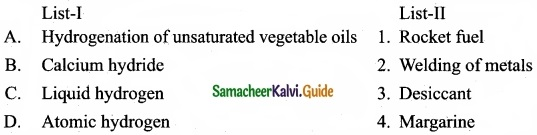Question 5.
Lithium shows diagonal relationship with ………………………
(a) Sodium
(b) Magnesium
(c) Calcium
(d) Ahuninium
(b) MagnesiumQuestion 6.
The variation of volume V, with temperature T, keeping pressure constant is called the coefficient of thermal expansion ie α = $$\frac{1}{V}$$ $$(\frac { \partial V }{ \partial T } )$$
(a) T
(b) 1/T
(c) P
(d) None of these
Solution: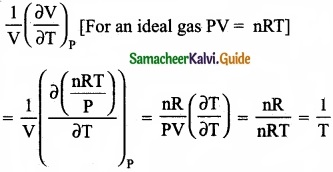(b) 1/T

Question 7.
Heat of combustion is always …………………….
(a) Positive
(b) Negative
(c) Zero
(d) Either positive or negative
(b) Negative

Question 8.
If in a mixture where Q = K, then what happens?
(a) The reaction shift towards products
(b) The reaction shift towards reactants
(c) Nothing appears to happen, but forward and reverse reactions are continuing at the same rate
(d) Nothing happens
(c) Nothing appears to happen, but forward and reverse reactions are continuing at the same rateQuestion 9.
Which one of the following gases has the lowest value of Henry’s law constant?
(a) N2
(b) He
(c) CO2
(d) H2
Solution:
Carbon dioxide; most stable gas and has lowest value of Henry’s Law constant.
(c) CO2

Question 10.
In the molecule OA = C = OB, the formal charge on OA, C and OB are respectively.
(a) – 1, 0, + 1
(b) + 1, 0, -1
(c) – 2, 0, + 2
(d) 0, 0, 0
Solution:Formal charge of OA/OB = NV – (Ne + $$\frac { N_{ b } }{ 2 }$$) = 6 – (4 + $$\frac{4}{2}$$) = 6 – 6 = 0
Formal charge of C = 4 – (0 + $$\frac{8}{2}$$) = 4 – 4 = 0
(d) 0, 0, 0

Question 11.
In the hydrocarbon CH3 – CH3 – CH = CH – CH2 – C = CH the state of hybridisation of carbon 1, 2, 3, 4 and 7 are in the following sequence.
(a) sp, sp, sp3, sp2, sp3
(b) sp2, sp, sp3, sp2, sp3
(c) sp, sp, sp2, sp, sp3
(d) None of these
(a) sp, sp, sp3, sp2, sp3Question 12.
Enzyme present in apple is ………………………..
(a) Polyphenol oxidase
(b) Polyphenol reductase
(c) Polyphenol
(d) Polyphenol hydrolase
(a) Polyphenol oxidase

Question 13.
The correct statement regarding the comparison of staggered and eclipsed conformations of ethane is ………………………….
(a) The eclipsed conformation of ethane is more stable than staggered conformation even though the eclipsed conformation has torsional strain.
(b) The staggered conformation of ethane is more stable than eclipsed conformation, because staggered conformation has no torsional strain.
(c) The staggered conformation of ethane is less stable than eclipsed conformation, because staggered conformation has torsional strain.
(d) The staggered conformation of ethane is less stable than eclipsed conformation, because staggered conformation has no torsional strain.
(b) The staggered conformation of ethane is more stable than eclipsed conformation, because staggered conformation has no torsional strain.Question 14.
In Finkelstein reaction, the mechanism followed is ……………………….
(a) SN1
(b) E1
(c) E2
(d) SN2
(d) SN2

Question 15.
Which sequence for greenhouse gases is based on GWP?
(a) CFC > N2O > CO2 > CH4
(b) CFC > CO2 > N2O > CH4
(c) CFC > N2O > CH4 > CO2
(d) CFC > CH4 > N2O > CO2
(c) CFC > N2O > CH4 > CO2

PART – II

Answer any six questions in which question No. 24 is compulsory. [6 × 2 = 12]

Question 16.
Avogadro number is the number of atoms present in one mole of an element or number of molecules present in one mole of a compound. The value of Avogadro number (N) = 6.023 × 1023.Question 17.
Explain the meaning of the symbol 4P. Write all the four quantum numbers for these electrons?
4f2: It means that the element has 2 electrons in outermost 4f shell.
Quantum number values are,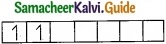n = principal quantum number = 4
l = azimuthal quantum number = 3
m = magnetic quantum number = – 3, -2
s = spin quantum number = +$$\frac{1}{2}$$, –$$\frac{1}{2}$$

Question 18.
Is the definition given below for ionization enthalpy is correct?
“Ionization enthalpy is defined as the energy required to remove the most loosely bound electron from the valence shell of an atom”?
No, It is not correct. Thq accurate and absolute definition is as follows:
Ionization energy is defined as the minimum amount of energy required to remove the most loosely bound electron from the valence shell of the isolated neutral gaseous atom in its ground state.Question 19.
What are the uses of calcium hydroxide?
Calcium hydroxide is used

1. In the preparation of mortar, a building material.
2. In white wash due to its disinfectant nature.
3. In glass making and tanning industry.
4. For the preparation of bleaching powder and for the purification of sugar.

Question 20.
30.4 kJ is required to melt one mole of sodium chloride. The entropy change during melting is 28.4 JK-1 mol-1. Calculate the melting point of sodium chloride?
Given:
∆Hf = 30.4 kJ = 30400 J mol-1
∆Sf (NaCl) = 28.4 kJ-1 mol-1
Tf = ?
∆Sf = $$\frac{\Delta \mathrm{H}_{\mathrm{f}}}{\Delta \mathrm{T}_{\mathrm{f}}}$$; Tf = $$\frac{\Delta \mathrm{H}_{\mathrm{f}}}{\Delta \mathrm{S}_{\mathrm{f}}}$$
Tf = $$\frac{30400 \mathrm{J} \mathrm{mol}^{-1}}{28.4 \mathrm{JK}^{-1} \mathrm{mol}^{-1}}$$ = 1070.4 KQuestion 21.
How is a gas-solution equilibrium exist?
When a gas dissolves in a liquid under a given pressure, there will be an equilibrium between gas molecules in the gaseous state and those dissolved in the liquid.
Example: In carbonate beverages the following equilibrium exists.
CO2(g) ⇄ CO2 (solution).

Question 22.
What type of hybridisations are possible in the following geometeries?

1. Octahedral
2. Tetrahedral
3. Square planar

1. Octahedral geometry is possible by sp3d2 (or) d2sp3 hybridisation.
2. Tetrahedral geometry is possible by sp3 hybridisation.
3. Square planar geometry is possible by dsp2 hybridisation.Question 23.
How will you prepare Lassaigne’s extract?
Lassagine’s extract preparation:

1. A small piece of Na dried by pressing between the folds of filter paper is taken in a fusion tube and it is gently heated. When it melts to a shining globule, a pinch of organic compound is added.
2. The tube is heated till reaction ceases and become red hot. Then it is plunged in 50 ml of distilled water taken in a china dish and the bottom of the tube is broken by striking it against the dish.
3. The contents of the dist is boiled for 10 minutes and then it is filtered. The filtrate is known as Lassaigne’s extract.Question 24.
Complete the reactions and identify the products?PART – III

Answer any six questions in which question No. 32 is compulsory. [6 × 3 = 18]

Question 25.
The balanced equation for a reaction is given below 2x + 3y → 41 + m
When 8 moles of x react with 15 moles of y, then

1. Which is the limiting reagent?
2. Calculate the amount of products formed.
3. Calculate the amount of excess reactant left at the end of the reaction.

2x + 3y → 41 + m
1. 2x reacts with 3y to give products.
8x reacts with 15y means, y is the excess because 8 moles of x should react with 4 × 3y = 12y moles of y to give products.
In this reaction 15y moles are used.
Therefore, 3 moles of y is excess and x is the limiting agent.

2. When 8 moles of x react with 12 moles of y, the product formed will be 4 × 41 i.e. 161 and 4m as product.
8x+ 12y → 161 + 4m

3. At the end of the reaction, the excess reactant left is 3 moles of y.Question 26.
Which would you expect to have a higher melting point, magnesium oxide or magnesium fluoride? Explain your reasoning.

1. Magnesium oxide has very strong ionic bonds as compared to magnesium fluoride.
2. Mg2+ and O2- have charges of +2 and -2, respectively.
3. Oxygen ion is smaller than fluoride ion.
4. The smaller the ionic radii, the smaller the bond length in MgO and the bond is stronger than MgF2.
5. Due to more strong bond nature in MgO, it has high melting point than MgF2.Question 27.
A sample of solid KClO3 (potassium chlorate) was heated in a test tube to obtain O2 according to the reaction
2KClO3(s) → 2KCl + 3O2
The oxygen gas was collected by downward displacement of water at 295 K. The total pressure of the mixture is 772 mm of Hg. The vapour pressure of water is 26.7 mm of Hg at 300K. What is the partial pressure of the oxygen gas?Question 28.
List the characteristics of entropy?
Characteristics of entropy:

• Entropy is a thermodynamic state function that is a measure of the randomness or disorderliness of the system.
• In general, the entropy of gaseous system is greater than liquids and greater than solids. The symbol of entropy is S.
• Entropy is defined as “for a reversible change taking place at a constant temperature (T), the change in entropy (∆S) of the system is equal to heat energy absorbed or evolved (q) by the system divided by the constant temperature (T).
$$\Delta S_{\mathrm{sys}}=\frac{q_{\mathrm{rev}}}{T}$$
• If heat is absorbed, then ∆S is positive and there will be increase in entropy. If heat is evolved, ∆S is negative and there is a decrease in entropy,
• The change in entropy of a process represented by ∆S and is given by the equation,
∆Ssys = Sf – Si
• If Sf > Si, ∆S is positive, the reaction is spontaneous and reversible.
If Sf < Si, ∆S is negative, the reaction is non-spontaneous and irreversible.
• Unit of entropy: SI unit of entropy is J K-1.Question 29.
Derive the values of KC and KP for the synthesis of HI.
H2(g) + I2(g) ⇄ 2HI(g)
Let us consider the formation of HI in which V moles of hydrogen, ‘b’ moles of iodine gas are allowed to react in an container of volume ‘V’.
Let ‘x’ moles of each of H2 and I2 react together to form 2x moles of HI.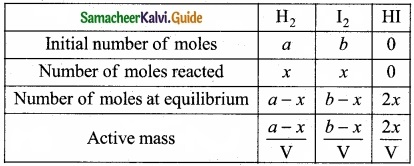Applying mass of action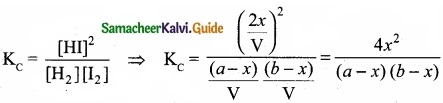Caluculation of KP: KP = KC.RT∆ng
Here ∆ng = np – nr = 2 -2 = 0
Hence, KP = KC
KP = $$\frac{4 x^{2}}{(a-x)(b-x)}$$Question 30.
Describe the classification of organic compounds based on their structure?
Classification of organic compounds based on the structure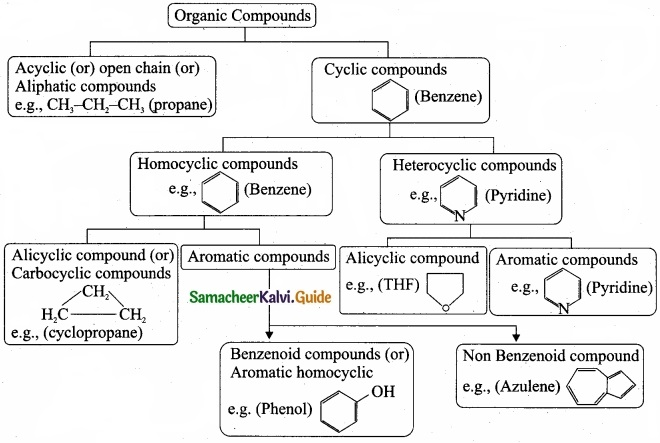Question 31.
For the following bond cleavages use curved-arrows to show the electron flow and classify each as homolytic or heterolytic fission. Identify reactive intermediate produced as free radical, carbocation and carbanion?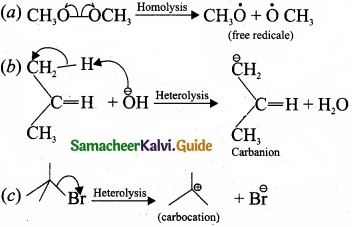Question 32.
An alkyl halide with molecular formula C6H12Br on dehydrohalogenation gave two isomeric alkenes X and,Y with molecular formula C6H12. On reductive ozonolysis, X and Y gave four compounds CH3COCH3, CH3CHO, CH3CH2CHO and (CH3)2 CHCHO. Find the alkyl halide?

1. C6 H13 Br is 3 – Bromo – 4 – methylpentane.2. 3 – Bromo – 4 – methylpentane on dehydration give two isomers X and Y as follows: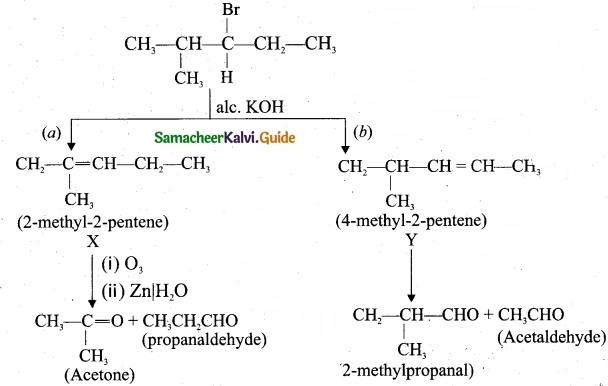Therefore C6H13 Br is 3 – Bromo – 4 – methylpentaneQuestion 33.
What do you mean by ozone hole? What are its consequences?
Depletion of ozone layer creates some sort of holes in the blanket of ozone which surrounds us in the atmosphere and this is known as ozone hole.

1. With the depletion of the ozone layer, UV radiations filters into the troposphere which leads to ageing of skin, cataract, sunburn, skin cancer etc.
2. By killing many of the phytoplanktons, it can damage the fish productivity.
3. Evaporation rate increases through the surface and stomata of leaves which can decrease the moisture content of the soil.

PART – IV

Answer all the questions. [5 × 5 = 25]

Question 34 (a).
(I) Balance the following equations by ion electron method?
KMnO4 + SnCl2 + HCl → MnCl2 + SnCl4 + H2O + KCl
(II) Boric acid, H3BO3 is a mild antiseptic and is often used as an eye wash. A sample contains 0.543 mol H3BO3. What is the mass of boric acid in the sample?

[OR]

(b)
(I) How many unpaired electrons are present in the ground state of Fe3+ (z = 26), Mn2+ (z = 25) and argon (z = 18)?
(II) Explain about the significance of de Broglie equation?
KMnO4 + SnCl2 + HCl → MnCl2 + SnCl4 + H2O + KCl
Oxidation half reaction: (loss of electron)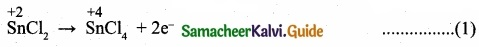Reduction of halfa reaction: (gain of electron)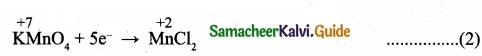Add H2O to balance oxygen atomsAdd Hcl to balance hydrogen atoms
KMnO4 + 5e + 8 HCl → MnCl2 + 4H2O …………………. (4)

To equalize the number of electrons equation (1) × 5 and equation (2) × 2(II) Molecular mass of H3BO3 = (1 × 3) + (11 × 1) + (16 × 3) = 62
Boric acid sample contains 0.543 mole.
Mass of 0.543 mole of Boric acid = Molecular mass x mole
= 62 × 0.543
= 33.66 g

[OR]

(b) (I) Fe → Fe3+ + 3e
Fe (Z = 26)
Fe3+ = number of electrons = 23
1s2 2s2 2p6 3s2 3p6 3d6 4s2 for Fe atom.
1s2 2s2 2p6 3s2 3p6 3d5 for Fe3+ ion.
So, it contain 5 unpaired electrons.
Mn (Z = 25) Electronic configuration is
1s2 2s2 2p5 3s2 3p6 4s2 3d5
Mn → Mn2+ + 2e
Number of unpaired electrons in Mn2+ = 5
Ar (Z = 18). Electronic configuration is
1s2 2s2 2p6 3s2 3p6.
All orbitals are completely filled. So, no unpaired electrons in it.

(II) Significance of de Broglie equation:

1. λ = $$\frac{h}{mv}$$ This equation implies that a moving particle can be considered as a wave and a wave can exhibit the properties of a particle.
2. For a particle with high linear momentum (mv) the wavelength will be so small and cannot be observed.
3. For a microscopic particle such as an electron, the mass is of the order of 10-31 kg, hence the wavelength is much larger than the size of atom and it becomes significant.
4. For the electron, the de Broglie wavelength is significant and measurable while for the iron ball it is too small to measure, hence it becomes insignificant.Question 35 (a).
(I) Mention any two anomalous properties of second period elements?
(II) Arrange Na+, Mg2+ and Al3+ in the increasing order of ionic radii. Give reason?

[OR]

(b)
(I) How do you expect the metallic hydrides to be useful for hydrogen storage?
(II) Write a note about ortho water and para water?
(a) (I) Anomalous properties of second period elements:

1. In the 1st group, lithium forms compounds with more covalent character while the other elements of this group form only ionic compounds.
2. In the 2nd group, beryllium forms compounds with more covalent character while the other elements of this family form only ionic compounds.

(II) Na+, Mg2+ and Al3+ are isoelectronic cations.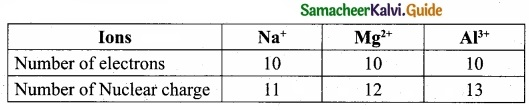The cation with the greater positive charge will have a smaller radius because of the greater attraction of the electrons to the nucleus. Hence the increasing order of ionic radii is, $$\mathbf{r}_{\mathrm{Na}^{+}}$$, > $$\mathbf{r}_{\mathrm{Mg}^{2+}}$$, > $$\mathbf{r}_{\mathrm{Al}^{3+}}$$.

[OR]

(b)
(I) In metallic hydrides, hydrogen is adsorbed as H-atoms. Due to the adsorption of H atoms the metal lattice expands and become unstable. Thus, when metallic hydride is heated, it decomposes to form hydrogen and finely divided metal. The hydrogen evolved can be used as fuel.

(II)
1. Water exists in space in the interstellar clouds, in proto-planetary disks, in the comets and icy satellites on the solar system, and on the Earth.
2. In particular, the ortho-to-para ratio (OPR) of water in space has recently received attention. Like hydrogen, water can also be classified into ortho – H2O and para – H2O, in which the directions are antiparallel.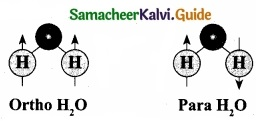3. At the temperature conditions of the Earth (300 K), the OPR of H2O is 3.
4. At low temperatures below (< 50 K) the amount of para – H2O increases. It is known that the OPR of water in interstellar clouds and comets has more para – H2O (OPR = 2.5) than on Earth.Question 36 (a).
Derive the values of critical constants from the Van der Waals constants?

[OR]

(b) Derive the values of Kp and KC for dissociation of PCl5?
(a) Derivation of critical constants from the Van der Waals constants:
Van der Waals equation is,
$$\left(P+\frac{a n^{2}}{V^{2}}\right)$$ (V – nb) = nRT for 1 mole.
From this equation, the values of critical constant PC, VC and TC are derived in terms of a and b the Vander Waals constants.The above equation (4) is an cubic equation of V, which can have three roots. At the critical point, all the three values of V are equal to the critical volume Vc. i.e. V = VC
i.e; V = VC
V – VC = 0 ………………… (5)
(V – VC)3 ………………….. (6)
(V3 – 3VCV2) + 3VC2V – VC3 …………………. (7)
As the equation (4) is identical with equation (7), comparing the ‘V’ terms in (4) and (7),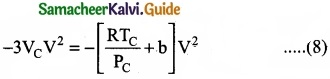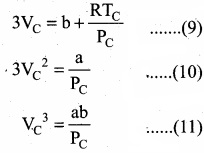Divide equation (11) by (10)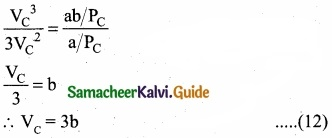When equation (12) is substituted in (10)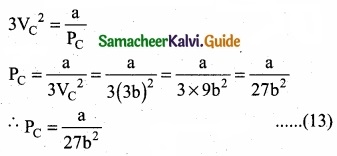Substituting the values of VC and PC in equation (9)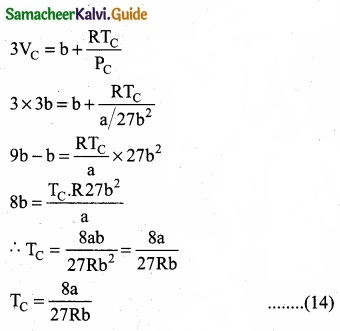Critical constants a and b can be calculated rising Vander Waals constants as follows: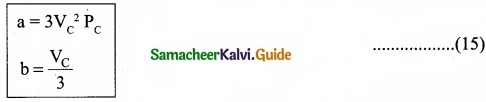(b) Consider that V moles of PCl5is taken in container of volume‘V’
Let x moles of PCl5 be dissociated into x moles of PCl3 and x moles of Cl2.Applying law of mass action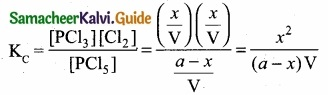Kp caluculation: Kp = KC. $$\mathrm{RT}^{\mathrm{An}}$$; ∆ng = 2 – 1 = 1
We know that PV = nRT
RT = $$\frac{PV}{n}$$
Where ‘n’ is the total number of moles at equilibrium
n = a – x + x + x = a + x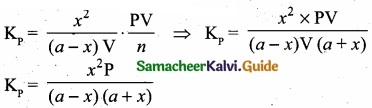Question 37 (a).
(I) Solubility of a solid solute in a liquid solvent increases with increase in temperature. Justify this statement?
(II) Explain how non-ideal solutions shows positive deviation from Raoult’s law?

[OR]

(b)
(I) How will you distinguish between electrophiles and nucleophiles?
(II) Complete the following reactions and identify the products?
(a)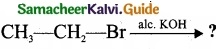(b)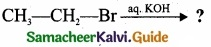(a) (I) When the temperature is increased,the average kinetic energy of the molecules of the solute and the solvent increases. The increase in the kinetic energy facilitates the solvent molecules to break the intermolecular attractive forces that keep the solute molecules together and hence the solubility increases.
(II)

1. Let us consider the positive deviation shown by a solution of ethyl alcohol and water
2. In this solution, the hydrogen bonding interaction between ethanol and water is weaker than those hydrogen bonding interactions amongst themselves (ethyl alcohol-ethyl alco¬hol and water-water interaction).
3. This results in the increased evaporation of both components from the aqueous solution of ethanol.
4. Consequently, the vapour pressure of the solution is greater than the vapour pressure
predicted by Raoult’s law.
5. Here, the mixing process is endothermic i.e., DHmixing > 0 and there will be a slight increase in volume (DVmixing > 0)
6.(b)
(I)
Electrophiles:

1. They are electron deficient.
2. They are cations.
3. They are lewis acids.
4. Accept an electron pair.
5. Attack on electron rich sites.

Nucleophiles:

1. They are electron rich.
2. They are anions.
3. They are lewis bases.
4. Donate an electron pair.
5. Attack on electron deficient sites.

(II)
(a)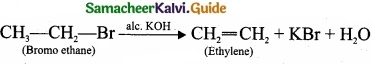(b)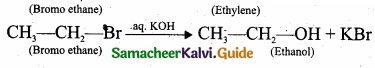Question 38 (a).
(I) Is it possible to prepare methane by Kolbe’s electrolytic method?
(II) Explain how 2-butyne reacts with
(a) Lindlar’s catalyst
(b) Sodium in liquid ammonia.

[OR]

(b) (I) Discuss the aromatic nucleophilic substitution reactions of chlorobenzene?
(II) CCl4 > CHCl3 > CH2C12 > CH3Cl is the decreasing order of boiling point in haloalkanes. Give reason?
(a) (I) Kolbe’s electrolytic method is suitable for the preparation of symmetrical alkanes, that is alkanes containing even number of carbon atoms. Methane has only one carbon, hence it cannot be prepared by Kolbe’s electrolytic method.
(II) (a) 2-butyne reacts with Lindlar’s catalyst: 2-butyne can be reduced to cis – 2 butene using CaCO3 supported in Pd -metal partially deactivated with sulphur.
This reaction is stereo specific giving only the cis – 2 – butene.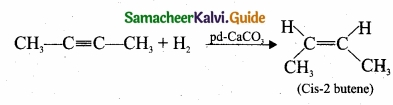(b) 2-butyne reacts with sodium in liquid ammonia:
2-butyne can also be reduced to trans – 2 – butene using sodium in liquid ammonia. This reaction is stereospecific giving only the trans – 2 – butene.[OR]

(b) (I) Aromatic nucleophilic substitution reactions:
Dow’s process:

1.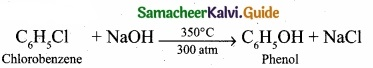2.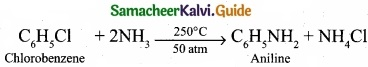3.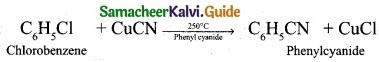(II) The boiling point of chloro, bromo and iodoalkanes increases with increase in the number of halogen atoms. So the correct decreasing order of boiling point of haloalkanes is:
CCl4 > CHCl3 > CH2Cl2 > CH3Cl.